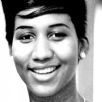Let It Be Chords

Aretha Franklin

Difficulty:Easy

by ARICALLet It Be

Year: 1970 - Album: This Girl's in Love with You

(John Lennon and Paul McCartney)

Key:  CLet It Be Key A#A#(one step down)
Let It Be Key BB(half step down)
Let It Be Key CC(original key)
Let It Be Key C#C#(half step up)
Let It Be Key DD(one step up)
Intro: C G Am F C G F C Dm C

C                  G                       Am               F
When I find myself in times of trouble, Mother Mary comes to me
C                              G              F  C   Dm   C
Speaking words of wisdom, let it be
C                         G                  Am                        F
And in my hour of darkness, she is standing right in front of me
C                              G               F  C  Dm   C
Speaking words of wisdom, Let it be
G     Am              G               F            C
Let it be,      let it be,     let it be,     let it be
G              F  C  Dm  C
Whisper words of wisdom, let it be
C              G              Am          F
And when the broken hearted people, living in the world agree
C                 G              F  C   Dm   C
There will be an answer, let it be
C              G                 Am          F
But though they may be parted, there is still a chance that they may see
C             G              F  C/E   Dm   C
There will be an answer, let it be
Am          G              F           C
Let it be, let it be,   let it be,    let it be
G                C              F  C/E   Dm    C
There will be an answer, let it be
G     Am              G               F            C
Let it be,      let it be,     let it be,     let it be
G              F  C  Dm  C
Whisper words of wisdom, let it be
G     Am              G               F            C
Let it be,      let it be,     let it be,     let it be
G              F  C  Dm  C
Whisper words of wisdom, let it be
C          G                 Am     F
And when the night is cloudy, there is still a light that shines on me
C                   G                  F  C   Dm   C
Shine on till tomorrow, let it be
C                            G                 Am                            F
I wake up to the sound      of music, Mother Mary comes    to me
C                              G              F  C  Dm   C
Speaking words of wisdom, let it be
G     Am              G               F            C
Let it be,      let it be,     let it be,     let it be
G              F  C  Dm  C
Whisper words of wisdom, let it be
G     Am              G               F            C
Let it be,      let it be,     let it be,     let it be
G              F  C  Dm  C
Whisper words of wisdom, let it be

Interlude: F C Dm C F Dm C G Am F C G F C Dm C G Am F C G F C Dm C

C          G                      Am     F
And when the night is cloudy, there is still a light that shines on me
C                   G                  F  C   Dm   C
Shine on till tomorrow, let it be
C                             G                 Am                            F
I wake up to the sound      of music, Mother Mary comes    to me
C                              G              F  C  Dm   C
Speaking words of wisdom, let it be
G     Am              G               F            C
Let it be,      let it be,     let it be,     let it be
G              F  C  Dm  C
Whisper words of wisdom, let it be
G     Am              G               F            C
Let it be,      let it be,     let it be,     let it be
G              F  C  Dm  C
Whisper words of wisdom, let it be
G     Am              G               F            C
Let it be,      let it be,     let it be,     let it be
G              F  C  Dm  C
Whisper words of wisdom, let it beFull key step upHalf key step upHalf key step downFull key step downshow chordsYouTube Cliphide all tabsgo to top